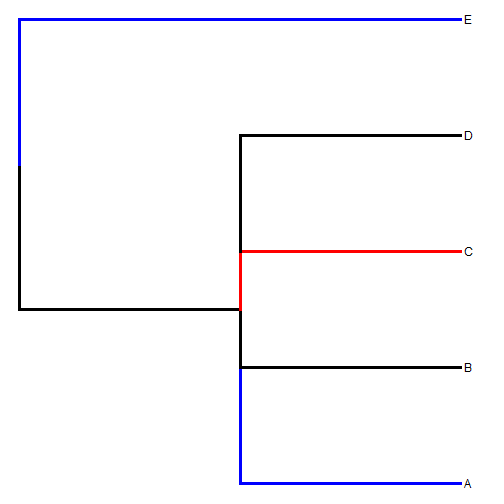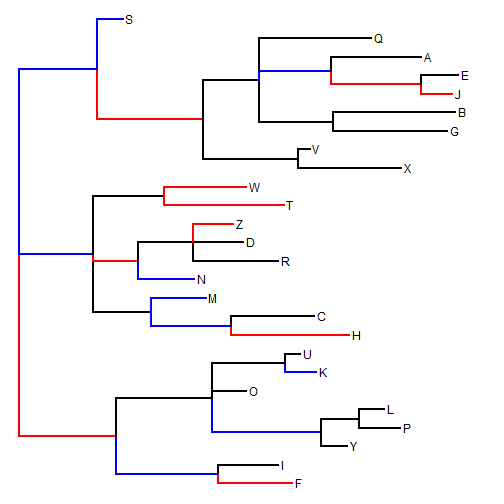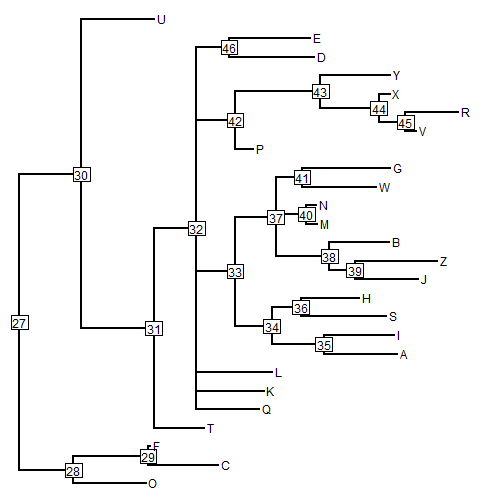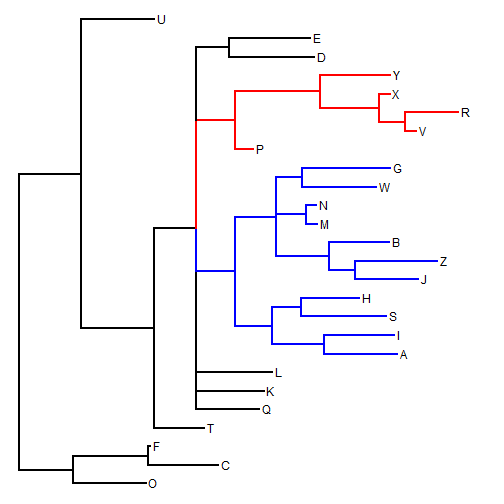## Saturday, September 5, 2015

### More on splitting vertical line colors by the mapped states on daughter edges

I just posted an update to the phytools function `splitEdgeColor` which allows a user plotting an object of class `"simmap"` in which the mapped character changes state exactly at one more nodes, to split the color of the vertical edges in a square phylogram to split colors according to the differing states of the two daughter edges (if they differ).

This update tells R what to do in the case of multifurcating nodes. What I decided is that the line segment between each daughter edge should be the color of the daughter edge north of that edge for daughters north of the parent edge; and vice versa for daughters south of the parent edge.

To clarify what that means, we can try the following 'toy' example:

``````library(devtools)
install_github("liamrevell/phytools",quiet=TRUE)
``````
``````library(phytools)
tree<-paintBranches(tree,1,"b","a")
tree<-paintBranches(tree,3,"c","a")
tree<-paintBranches(tree,5,"b")
colors<-setNames(c("black","blue","red"),c("a","b","c"))
plot(tree,colors,split.vertical=TRUE,lwd=3)
``````Here are some other examples of how it looks:

``````set.seed(100)
tree<-rtree(n=26,tip.label=LETTERS)
tree\$edge.length[(tree\$edge.length<0.25)*(tree\$edge[,2]>Ntip(tree))==1]<-0
di2multi(tree)->tree
b<-sample(tree\$edge[,2],10)
c<-sample(setdiff(tree\$edge[,2],b),10)
tree<-paintBranches(tree,b,"b","a")
tree<-paintBranches(tree,c,"c")
plot(tree,colors,split.vertical=TRUE)
``````Or when we have used `paintSubTree`:

``````tree<-rtree(n=26,tip.label=LETTERS)
tree\$edge.length[(tree\$edge.length<0.25)*(tree\$edge[,2]>Ntip(tree))==1]<-0
di2multi(tree)->tree
plotTree(tree,node.numbers=TRUE)
````````````tree<-paintSubTree(tree,33,"b","a",stem=TRUE)
tree<-paintSubTree(tree,42,"c","a",stem=TRUE)
tree<-paintSubTree(tree,41,"b",stem=TRUE)
plot(tree,colors,split.vertical=TRUE)
``````That's it.

#### 1 comment:

1.Hi Liam, is it possible that I color the tree edges by a subset of species names list (the names are identical with those on the tree)?

Thanks!

Miao

Note: due to the very large amount of spam, all comments are now automatically submitted for moderation.